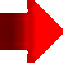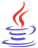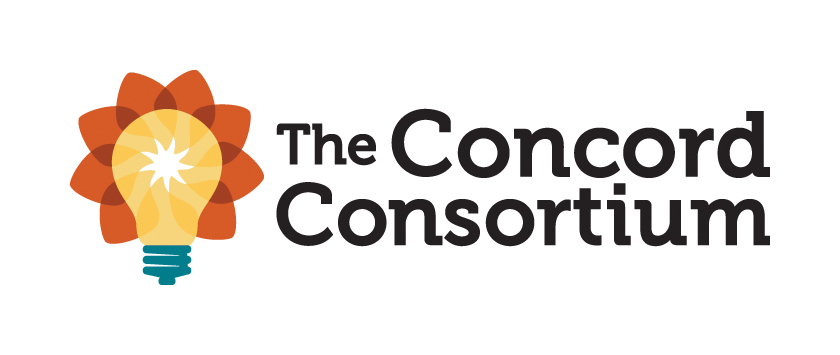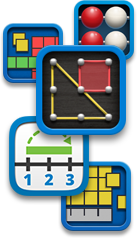# Math Manipulatives: Virtual Manipulatives on the WebMath Manipulatives contains three pages of resources:

Math Manipulatives (Page 1 of 3): Read a short essay about virtual manipulatives and their role in learning mathematicsVirtual Manipulatives on the Web (Page 2 of 3):

Math Manipulatives (Page 3 of 3): Calculators and PDA resources, including calculator tutorials, activities, software enhancements, and calculator apps for mobile devices## Virtual Manipulatives on the Web#### Technology Requirements:JAVA: You might need to download Java, a free plug-in to interact with virtual manipulatives.  Note: See Java's Help information on how to enable Java in your web browser, if an applet does not run after an installation.

Note: Newer versions of virtual manipulatives are written in HTML5.

Analyze Math by A. Dendane (United Arab Emirates University) has an extensive collection of applets in the section called Math Software.  Learners can explore and gain deep understanding of topics in mathematics including calculus, precalculus, geometry, trigonometry and statistics.  There is also a section for Interactive HTML5 Math Web Apps for Mobile Learning.

Annenberg Learner: Geometry 3-D Shapes is an interactive intended for grades 6-8, although other grades can benefit.  Students can interactively learn about three-dimensional shapes, calculate surface area and volume, and discover some of the mathematical properties of shapes. It comes with a glossary, tutorials, and an interactive test.

CalcPlot3D is a Java applet from the Mathematical Association of America Mathematical Sciences Digital Library Loci Resources, which provides an exploration environment for multivariable calculus.

Complex Numbers--An Interactive Introduction to Complex Numbers includes two Java applets, explanatory text, and exercises for exploring basic principles of complex numbers (e.g., basic arithmetic, conjugation, powers, roots).  Both applets allow users to "input complex numbers in either cartesian or exponential form and display them in vector form."  Content is posted within the Mathematical Association of America Mathematical Sciences Digital Library Loci Resources.Concord Consortium STEM resources: Mathematics.  Each virtual manipulative is accompanied by an activity, teacher lesson plan, and assessment.  Standards for the resource are also provided (e.g., NCTM,  Common Core standards).  Searches can be narrowed by elementary, middle, high school, or higher education.

Didax 19 virtual manipulatives for math include unifix cubes, ten frames, number lines, Rekenrek beads, base ten blocks, dice, spinners, algebra tiles, and more.  Instructions, how-to videos, and activities are included.

DreamBox Learning has over 40 free virtual manipulatives for K-8 math available on its website that can also be used with any whiteboard.  They help learners engage with number sense, addition, subtraction, multiplication, division, geometry, algebra, functions, and proportional reasoning concepts.Erathothenes' Sieve is an ancient method for calculating prime numbers, explained in this video posted at Khan Academy.  The algorithm is still used today.  Prime Numbers Before Calculator from factors-of.com will show you the prime numbers before a given number until 10,000.  Learn more about the largest known prime at the Great Internet Mersenne Prime Search.  Found in December 2018, the largest prime is 282,589,933-1.  It has 24,862,048 digits!  Then learn more about this discovery in GIMPS Discovers Largest Known Prime Number

Euclid's Elements Dr. Dave Joyce of Clark University in Massachusetts brings all 13 books of the Elements alive using a Java geometry applet to illustrate definitions and postulates.

ExploreLearning.com is a subscription based set of over 400 virtual manipulatives (simulations of math and science concepts) for grades 3-12, which are called "Gizmos."  However, you can sign up for a free account for unlimited use of a free list of several Gizmos.  ExploreLearning Gizmos help students develop critical thinking skills and conceptual understanding.  Learning materials include teacher guides, student exploration sheets to guide scaffolded lessons with vocabulary, prior knowledge questions, and more.  Search by subject, grade band, or topic.  The series is correlated to Common Core and state standards, major textbooks, and includes assessment and real time reporting. See the resource catalog.

Floorplanner "an online CAD (Computer Aided Design) system specifically developed for bringing good-looking, complex floor plans within reach of everyone" (About Us section).  Educators might consider using it with learners in upper elementary grades through high school for creating 2D and 3D plans--great for geometry studies.  Create an entire house with interactive floor plans.  A free version is available for personal use.

GeoGebra is free, open-source, dynamic mathematics software that joins geometry, algebra, statistics, and calculus. You can do constructions with points, vectors, segments, lines, conic sections as well as functions and change them dynamically afterwards.  You can enter equations and coordinates directly. GeoGebra has the ability to deal with variables for numbers, vectors and points, finds derivatives and integrals of functions and offers commands like Root or Extremum.  It received several international awards, including the European and German educational software awards. In Dynamic Mathematics with GeoGebra, authors Markus Hohenwarter, principle developer of the software, and Judith Preiner (2007) said, "You can use GeoGebra both as a teaching tool and to create interactive web pages for students from middle school up to college level. Specifically designed for educational purposes, GeoGebra can help you to foster experimental, problem-oriented and discovery learning of mathematics" (Abstract section).  Additional resources for using GeoGebra include:

• GeoGebra's Blog
• Exploring Geometric Transformations in a Dynamic Environment, posted within the Mathematical Association of America Mathematical Sciences Digital Library Loci Resources.  You'll find eight activities and virtual manipulatives related to teaching reflections (also including reflections with parallel lines, intersecting lines, and concurrent lines), rotations, translations, and glide-reflections to middle and high school learners.
• GeoGebra: Virtual Manipulatives includes twelve ready-to-use virtual manipulatives by Duane Habecker, which are most suitable for learners in elementary grades.
• GeoGebra: Elementary School Math: 300+ Resources authored by the GeoGebra Team includes interactives to explore concepts and supporting videos.
• GeoGebra: Middle and High School Math: 2200+ Resources authored by the GeoGebra Team includes interactives to explore concepts in multiple subjects and supporting videos.
• GeoGebra: Calculus Applets by Marc Renault of Shippenburg University includes his excellent collection of applets on limits, differentiation, applications of the derivative, and integrals.  Applets are written using HTML5.
• GeoGebra: Calculus Applets by Tim Brzezinski is a collection of applets that relate to limits and continuity, derivatives, integrals, differential equations, polar coordinates, parametric equations, sequences and series, multivariable calculus, and more.
• GeoGebra: Calculus I, II, and III lessons and applets by Tuyetdong Phan-Yamada, a math department lecturer at California State University, Los Angeles.  Phan-Yamada also has lessons and applets for discrete math, differential equations, statistics, finite math, algebra (linear, beginning, intermediate, college) and geometry.

Geometry Applet Gallery from Saltire Software contains some excellent applets, which are easy to use and suitable for illustrating several theorems presented in a high school or post-secondary geometry course.  Basic Geometry relates to angles in parallel lines, circular configurations, Pythagorean triples and incircles. Students can drag points to explore concepts.  Four triangle calculators are presented.  By entering SSS, SAS, or ASA, students can see the unique triangle that is formed, but if SSA is entered the resulting triangle is not unique (two are possible) or no triangle is possibly formed with given information.  Some advanced geometrical configurations are explored.  Saltire's work has been assisted with a number of National Science Foundation grants.

Geometry Playground is a free Java applet from the Mathematical Association of America Mathematical Sciences Digital Library Loci Resources, which enables "ruler and compass" constructions in "Euclidean, Spherical, Projective, Hyperbolic, Manhattan and Conical geometries" (Description section).  Potential classroom activities are included.

Illuminations Interactives from the National Council of Teachers of Mathematics (NCTM) contain virtual manipulatives and applets identified by grade band to investigate various concepts within NCTM identified strands and Common Core Math Standards searchable by grade level.  Apps for mobile devices are also among interactives.

Interactivate, which is a project of The Shodor Education Foundation, Inc., includes a set of interactive JAVA-based tools to support courseware in science and math.  Activities are divided by grade-band (3-5, 6-8, 9-12, and undergraduate).  Some activities allow students to keep score to monitor their progress, and are listed under assessments.  Activities that use the tools support Common Core standards and you can search by standards to find specific resources addressing those.  Browse by topic, audience, type (activity, lesson, discussion), or subject (number and operations, geometry, algebra, probability, statistics, modeling, discrete, and other).  A math dictionary of terms used within the site is included.  Interactivate has also aligned its activities to several textbook series (e.g., Connected Math, Math Thematics, Mathematics in Context, and MathScape).Interactive Mathematics promotes learning math while you play with it!  Primarily for grade 8 algebra through upper secondary and college level students; math lessons include topics in algebra, complex numbers, counting & probability, logarithms, trigonometry, money math, plane analytic geometry, and graphing.  Calculus includes differentiation, integration, and higher topics in calculus.  Lectures and full examples are provided.  There's also a section on math art in code.  A complete list of interactive graphs, calculators, and other manipulatives is available.  The site is the work of Murray Bourne.

Key Curriculum Press JavaSketchpad has several applets for use with secondary math students and above.  In the Sketchpad Gallery you will find multiple demonstrations from geometry (e.g., centroid, Pythagorean theorem), trigonometry and analytic geometry (e.g., sine wave and conic sections), calculus (e.g., box volume and falling ladder), mechanics, and other explorations (e.g. least squares).

Larry Green's Applet Page has numerous applets within basic math, beginning algebra, intermediate and college algebra, statistics, and calculus.  This is well worth an investigation.

Law of Sines and Law of Cosines are two applets that allow you to input sides and angles per each law and then each applet calculates the missing sides and angles in a triangle.  Some practical applications of each law are also included.  These are hosted by the Department of Physics and Astronomy at Georgia State University.

Math Open Reference includes free content in algebra, geometry (plain, solid, coordinate), trigonometry, constructions, and calculus.  Its focus is on using interactive materials and animations to develop concepts.  There are numerous interactive applets with at least one accompanying nearly every concept.  This is highly recommended for visual learners and reinforcement for all.  Math Open Reference includes its alignment with the Common Core Math Standards and links to its resources related to those.

Mathenæum has numerous apps written in HTML5 for number, geometry, algebra, chance and data, and models, the latter of which is associated with arithmetic and algebra to "help develop understanding of the mathematics and processes underlying more formal mathematical representations."

MathsBot has an extensive collection of virtual manipulatives written in HTML5 and additional tools for teachers.

Math Doodles is by Daren Carstens, who is the developer of the award winning software Math Arena.  He offers several interactive puzzles and games for elementary learners to have fun.  But there is learning, too, as students practice addition, subtraction, money skills, and pattern matching (shape, number, color), for example.  Among current games are Connect Sums, Number Jump, Polyomino Shift,  Double Traits, Sum Stacker, Time Shuffle, and more.  Older learners also benefit, as students really need to think as they do these.

Math Education Page: Applets created by Henri Picciotto using GeoGebra.  You'll find applets for fractions, algebra, functions, and geometry designed to make "various mathematical ideas more visual, and more interactive."Math Learning Center Apps
Platform: available in two or more versions: iOS for Apple mobile devices, Chrome, Windows, and  Web apps which work on all modern browsers
Price: Free
Info: The Math Learning Center free virtual manipulative apps are based on visual models featured in Bridges in Mathematics K-5 and include the following:

• Fractions
• Pattern Shapes
• Money Pieces
• Number Frames
• Math Vocabulary Cards
• Geoboard for K-8
• Number Line
• Number Pieces (base-ten blocks)
• Number Pieces Basic
• Number Rack

Math Playground: Math Manipulatives:  This learning site for grades 1-6 includes virtual math manipulatives, such as a protractor, fraction bars, equivalent fractions, percents, a spinner for probability, function machine, pattern blocks, and a geoboard, and more.

Math Warehouse is "dedicated to dynamic Math lessons, demonstrations, and interactive activities." There are topics in algebra, geometry, trigonometry.  Worksheets are available for download, too.  The math manipulatives are created in HTML5 and can be used on an iPad.  There are applets for circles, triangles, lines, calculators, distance formula, midpoint, fractions, and so on.

HOT: Mathigon: Polypad includes a series of manipulatives that you can move onto the pad: Polygons, 3-D Solids (you can unfold them to see its net and rotate them), Number Tiles, Number Bars, Fraction Bars, Algebra Tiles, Pentominoes, Probability and Data, a Tangram, and more.

MathinSite Applets address pure and applied mathematics.  Several pure mathematics applets are suitable for middle and high school learners.  Some applets come with work sheets and theory sheets.  You'll find: Straight Line, Parabola, Cubic, Exponential Function, Circle, Ellipses and Circles,Trig Functions, Adding Sinusoids; Piecewise, Odd/Even & Periodic Functions; Complex Numbers, Maclaurin Series; Arithmetic, Geometric & Linear Sequences; and Differentiation.  The site is from Bournemouth University (UK).

National Library of Virtual Manipulatives for Interactive Mathematics contains manipulatives and associated online activities for each of the five strands identified in standards set forth by the NCTM.  This is an ongoing project of Utah State University and a must see site for grades preK-12.  Select a manipulative from the grade level you teach and an activity from one of the strands.

NCTM Interactives come with the activity, instructions, and questions for exploration.  The database is searchable.  Common Core standards are identified.

PBS LearningMedia includes free media resources searchable by grade level, standards, media type, and subject. Resources include video and audio segments, interactives, images, documents, lesson plans, productivity tools for teachers, self-paced lessons,and student-oriented activities. You'll find media from NOVA, Frontline, American Experience, and other public broadcasting and content partners.  The mathematics section features K-8 mathematics strands, and high school number and quantity, algebra, geometry, functions, statistics and probability.  Highly recommended.

PhET Interactive Simulations from the University of Colorado at Boulder has " an extensive suite of simulations to improve the way that physics, chemistry, biology, earth science and math are taught and learned.  The simulations are interactive tools that enable students to make connections between real life phenomena and the underlying science which explains such phenomena" (About PhET section). The simulations are free, and can be easily used in the classroom.  There are over 40 math simulations that can be also be filtered by elementary, middle, high school, and university.

Principles and Standards for School Mathematics Electronic Examples--interactive applets for investigating concepts from NCTM.  Examples are divided into grade bands.

• preK-2: Investigate patterns, triangles and properties of polygons, geometry and measurement; develop spatial skills by using tanagrams, learn estimation strategies
• 3-5: Communicate about math using games, use simulation software to investigate distance, rate, and time; investigate data
• 6-8: Visualize the concept of multiplication, investigate rate of change, length, perimeter, area, volume, congruence, similarity, and symmetry, and the Pythagorean Theorem
• 9-12: Explore vectors; use graphs, equations, and tables to investigate elimination of medicine from the body; explore inscribed figures, linear regression, and linear functions.

HOT: Robo Compass is an online app for geometric constructions, transformations, patterns and tesselations.  It works "exactly as you do using a physical straightedge, compass and protractor."  See the extensive colorful gallery of what students have produced.  Documentation is included.

Ruler Game: Learn to read a ruler.  You can set the degree of precision for practice and select from the standard English or Metric rulers.  Users click on the ruler for the location of the measurement to find.

Seeing Math by the Concord Consortium has eight free secondary math interactives written in Java to assist algebra learners in the study of functions.  "Each interactive provides a real-time connection between representations of the mathematics (symbolic, graphical, etc.), so that changes in one representation instantly cause changes in the other."  Interactives include a qualitative grapher, piecewise linear grapher, linear transformer, quadratic transformer, function analyzer, system solver, plop it and proportioner.  A user's guide, warm up exercise, frequently asked questions, and sample activity are provided.

Seeing Theory is a visual introduction to probability and statistics.  Multiple interactive visualizations include explanations and are provided in categories of basic probability, compound probability, distributions, statistical inference, and linear regression.  Students studying AP Statistics or introductory college statistics will value this site.Statistics: Are you teaching your students about statistics and survey research?  Read Statistics Every Writer Should Know by Robert Niles.  Learn about mean, median, percent, per capita, standard deviation, margin of error, data analysis, and more.  Link to sites for data sources and interactive help to select the right statistical test.

• Introduction to Descriptive Statistics by Jay Hill at the University of Illinois.
• Chi-Square by Amar Patel. The material in this lesson, which is correlated to NCTM standards for grades 9-12,  will help students to understand statistical terms such as fairness, expectation, significance, and chi-square.  Excel spreadsheet is needed.  Content could be used with grade 8 and above, however.  Cartoon characters add humor to the discussion.
• Tools:  Generate random numbers, sample sizes, charts and graphs, and perform statistical calculations using these tools:

Statistics: The Art and Science of Learning from Data (5th edition, 2020) is an introductory textbook on statistics with multiple web apps.  Apps are available online.  "Use the apps to construct graphs, obtain summary statistics, find probabilities under the normal distribution, get confidence intervals or fit linear regression models. Take screenshots of your analyses or download graphs as .png files" (Web Apps section).  There are YouTube videos to explain various statistical concepts and multiple data sets that can be downloaded.

Tabula for grades 5-12 has dynamic geometry (DG) features similar to those in other DG programs.  However, developer David Hartmann indicated it can be distinguished from them as follows: "1. It has a set of virtual manipulation tools for folding, cutting, and taping. This set is designed to help teachers guide and extend classroom activities involving paper, scissors, etc.  Many teachers are familiar with Michael Serra’s Patty Paper Geometry book in which properties of shapes are discovered by folding and working with paper in other ways.  Tabula can be used to model the steps in most of the activities.  2. It has a different approach to transformations that incorporates pushpins and animation.  This is particularly designed to help students struggling with visual/spatial skills.  3. It has a set of virtual instruments that model their real counterparts.  This includes a traditional compass, a safety compass, a ruler, and a protractor.  Unlike models commonly used on electronic whiteboards, these instruments can be used directly in constructions and proofs.  4. It’s easy to use.  The research suggests that DG software can be beneficial, but that in order to realize the benefits, a serious commitment to preparation and classroom time must be made.  Tabula requires less of a time commitment and many of the same benefits." (personal communication, November 4, 2011)

TeacherLED, produced by Spencer Riley, a teacher since 2002 in Birmingham, United Kingdom, "is designed to provide teachers with access to free high quality teaching resources for use on Interactive Whiteboards."  Math resources are categorized by algebra, data handling, number, shape/space/measure, general maths, and investigations.Thinking Blocks at Math Playground teaches students in grades 1-7 how to visualize and solve math word problems. Using interactive blocks and cubes, they create models that illustrate the underlying math concepts within the problems. Skills include addition, multiplication, fractions, ratios, and there is a modeling tool for students to develop strong problem solving skills.

Toy Theater has multiple virtual manipulatives for math: fractions, time, geometry, number charts, counters, place value, probability, money, graph, and more.

Utah Education Network K-12 Student Center features student interactives categorized by math topics for gradebands K-2, 3-6, and 7-12.Visual Polyhedra is an extensive collection of polyhedra within multiple categories, which allows viewers to move and spin each.  The site by David McCooey also includes relevant characteristics of each.  Also see the Polyhedra Viewer, a collection of 120 solids that you not only can look at, but move and spin.

Visual Calculus from the University of Tennessee Math Department at Knoxville is an excellent resource that includes tutorials, drills and programs for precalculus and calculus.  Animations, interactive pages, step-by-step solutions and illustrations are included for topics ranging from functions, graphing with technology, solving equations, and conic sections to limits and continuity, derivatives and applications, integration and applications, sequences and series.  As a veteran calculus teacher, Patricia Deubel highly recommends this site.Visual Fractions by Richard Rand is an online tutorial with instruction and interactive practice in identifying, renaming, comparing, and operating on fractions, including mixed numbers (add, subtract, multiply, and divide). All examples are modeled with number lines or circles.  This site helps students, primarily in grades 3-8, to picture fractions and operations on them.

Visual Math Learning is a free educational web site developed by W. Bateman, who indicates that the site features "an interactive on-line tutorial for teaching elementary mathematics and basic arithmetic for grades K-12 at the pre-algebra level. It is an instructional aid for parents, teachers, and educators of primary, elementary, and middle school students, as well as a resource for lesson plans, homework help, and home schooling math lessons. The tutorial includes games, puzzles, interactive diagrams, and computer animated virtual manipulatives that emphasize active learning concepts by visualization."

Walter Fendt's HTML5 Applets on Mathematics address arithmetic, plane geometry, trigonometry (visual demonstration of graphs of sine, cosine and tangent of an angle), vector calculation, analysis (e.g. app to introduce the derivative, 1st and 2nd derivatives), and complex numbers (arithmetic operations).  These are very engaging and illustrative of concepts.

WISE: Web Interface for Statistics Education from Claremont University includes interactive tutorials and applets.WisWeb is the website of the Freudenthal Institute for middle and high school education (students of 12 to 18 years old). The main focus of the site is applets.  Subjects include number sense, number and estimation, measurement, algebra and calculus, geometry, discrete math, statistics and probability.  The Freudenthal Institute is a Research Group on Mathematics Education located in the Netherlands.

Wolfram Demonstrations Project from Wolfram Research, Inc. is a collection of interactive illustrations "to illuminate concepts in science, technology, mathematics, art, finance, and a remarkable range of other fields" (About the Wolfram Demonstrations Project section). You can preview Demonstrations directly in your browser, but to fully interact with them, download the live versions and run them in the free Wolfram Player, if you don't already have Mathematica (Version 6 or higher) or Wolfram Player Pro. Educators might also wish to download the "Experience Mathematica in Education" brochure to learn how to instantly make lessons interactive and use these Demonstrations to help you visualize classroom concepts.  Math demonstrations are provided for grades K-2, 3-5, middle school, and high school by subject, including calculus.  Demonstrations (manipulatives) are also aligned to Common Core standards.  Highly recommended.See related topics:  Math Resources and Standardized Test Preparation.